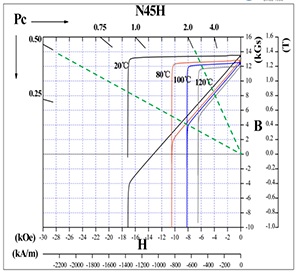# Magnetic Losses

Issuing time:2020-04-30 11:28

Reversible losses are normal behavior for all magnetic materials. As the remanence of the all the magnetic materials decreases with increasing temperature, the temperature coefficient is negative, it is to be expected that the magnetic field of a magnet also will decrease with an increase of the temperature. However, this decrease of magnetic field is reversible, meaning that when the temperature returns to room temperature, the magnetic field will be the same as in the beginning.Table below shows typical values of temperature coefficients of remanence and coercivity for some selected materials. Please note that the given values represent an average value over the given temperature range. The value of the coefficients typically increase with increasing temperature.

To avoid irreversible losses is one of the main tasks of magnetic circuit design. Irrevesible losses takes place if the working point of a magnet goes over the ”knee” in the BH-curve. The figure below, showing demagnetization curves of NdFeB N45H at various temperatures and working lines (green dotted lines) of magnets with permeance coefficients of 0,5 and 2.0, illustrates the behaviour.In the case of the magnet with Pc = 0.5, the working line is above the knee at 80C but at the knee at 100C. So, in oder to avoid irreversible losses, the maximum working temperature for this magnet is 80C. However, if the permeance coefficient is 2.0, the working line is above the knee even at 120C, and the magnet can thus be used up to 120C.It is true that usually magnets with high coercivity can be used in higher temperatures. However, it must be noted that the design of the magnetic circuit, i.e. the working point or permeance coefficient, is equally important. Sintered NdFeB N45H, for example, maximum operation temperature of 120C is usually indicated. This, however, is correct only if the working point is high enough, as illustrated here.

Tel： 0086 (0)571 82875116
E-mail： hpmg@china-hpmg.com
The South Beach of 2nd Qianjiang Bridge, Xiaoshan 311231 Hangzhou, P.R. China